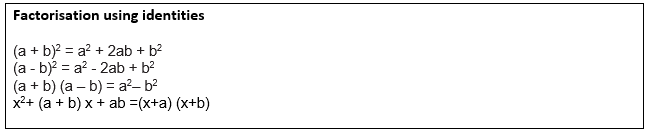# Class 8 Maths Exercise 14.2 Solutions

In this page we have Class 8 Maths Exercise 14.2 Solutions for Factorisation.This exercise has questions on Factorisation using identities.Free pdf download also available .Hope you like them and do not forget to like , social share and comment at the end of the page.

## Class 8 Maths Exercise 14.2 Solutions

Question 1
Factorize the following expressions.
(i) a² + 8a + 16
(ii) p² – 10 p + 25
(iii) 25m² + 30m + 9
(iv) 49y² + 84yz + 36z²
(v) 4x² – 8x + 4
(vi) 121b² – 88bc + 16c²
(vii) (l + m) ² – 4lm
(viii) a4 + 2a²b² + b4
We have to make use of following identities to factorize them
(a+b)2= a2 +b2 +2 ab
(a-b)2= a2 +b2 -2 ab
a2 –b2 = (a-b)(a+b)
(i) a² + 8a + 16
= a2 + 2×a× 4 +  42
So from first identity, it can be written as
=(a+4)2
(ii)  p² – 10 p + 25
= p2 -  2×p× 5 +  52
So from second identity, it can be written as
=(p-5)2
(iii) 25m² + 30m + 9
= (5m)2 + 2×5m× 3 +  32
So from first identity, it can be written as
=(5m+3)2
(iv) 49y² + 84yz + 36z²
= (7y)2 + 2×7y× 6z +  (6z)2
So from first identity, it can be written as
=(7y+6z)2
(v) 4x² – 8x + 4
= (2x)2 -  2×2x× 2 +  22
So from second identity, it can be written as
=(2x-2)2
= 4(x-1)2  taking common factor 2 out of square
(vi) 121b² – 88bc + 16c²
= (11b)2 - 2×11b× 4c +  (4c)2
So from second identity, it can be written as
=(11b-4c)2
(vii) (l + m) ² – 4lm
=l2 + m2 +2lm -4lm
= l2 + m2 -2lm
So from second identity, it can be written as
=(l-m)2
(viii) a4 + 2a²b² + b4
= (a2)2 + 2a²b²+(b2)2
So from first identity, it can be written as
=(a²+b²)²

Question 2
Factorize.
(i) 4p² – 9q²
(ii) 63a² – 112b²
(iii) 49x² – 36
(iv) 16x5 – 144x³
(v) (l + m) ² – (l – m) ²
(vi) 9x² y² – 16
(vii) (x² – 2xy + y²) – z²
(viii) 25a² – 4b² + 28bc – 49c²
We have to make use of following identities to factorize them
(a+b)2= a2 +b2 +2 ab
(a-b)2= a2 +b2 -2 ab
a2 –b2 = (a-b)(a+b)
(i) 4p² – 9q²
=(2p)2 –(3q)2
So from third identity, it can be written as
=(2p-3q)( 2p+3q)
(ii) 63a² – 112b²
= 7( 9a2 -16b2)
=7[ (3a)2 –(4b)2]
So from third identity, it can be written as
=7(3a-4b)( 3a+4b)
(iii) 49x² – 36
=(7x)2 –(6)2
So from third identity, it can be written as
=(7x-6)( 7x+6)
(iv) 16x5-144x3
= x³(16x²-144)
= x³(4x+12)(4x-12)
(v) (l + m) ² – (l – m) ²
= [ l+m +l-m][l+m-l+m]
=2l×2m
=4lm
(vi) 9x² y² – 16
= (3xy-4)(3xy+4)
(vii) (x² – 2xy + y²) – z²
=  (x-y)2 –z2   as (a-b)2= a2 +b2 -2 ab
Now as a2 –b2 = (a-b)(a+b)
= (x-y+z)(x-y-z)
(viii) 25a² – 4b² + 28bc – 49c²
Factorizing each tem
= (5a)2 –(2b)2 + 2×2b×7c –(7c)2
Rearranging the terms
=(5a)2 –[(2b)2 - 2×2b×7c +(7c)2]
Now as (a-b)2= a2 +b2 -2 ab
=(5a)2 –(2b-7c)2
=(5a-2b+7c)(5a+2b-7c)

Question 3
Factorize the expressions.
(i) ax² + bx
(ii) 7p² + 21q²
(iii) 2x³ + 2xy² + 2xz²
(iv) am² + bm² + bn² + an²
(v) (lm + l) + m + 1
(vi) y (y + z) + 9 (y + z)
(vii) 5y² – 20y – 8z + 2yz
(viii) 10ab + 4a + 5b + 2
(ix) 6xy – 4y + 6 – 9x
(i) ax² + bx
=x(ax+b)
(ii) 7p² + 21q²
=7(p2+3q2)
(iii) 2x³ + 2xy² + 2xz²
=2x(x²+y²+z²)
(iv) am² + bm² + bn² + an²
Rearranging the terms
= am²+ an²+ bm² + bn²
=a(m2 +n2) +b(m2 +n2)
=(a+b) (m2 +n2)
(v) (lm + l) + m + 1
=l(m+1) +1(m+1)
=(l+1)(m+1)
(vi) y (y + z) + 9 (y + z)
= (y+z)(y+9)
(vii) 5y² – 20y – 8z + 2yz
=5y(y-4) +2z(y-4)
=(5y+2z)(y-4)
(viii) 10ab + 4a + 5b + 2
=2a(5b+2) +1(5b+2)
=(2a+1)(5b+2)
(ix) 6xy – 4y + 6 – 9x
=2y(3x-2) +3(2-3x)
=2y(3x-2)-3(3x-2)
=(2y-3)(3x-2)

Question 4
Factorize.
(i) a4 – b4
(ii) p4 – 81
(iii) x4 – (y + z)4
(iv) x4 – (x – z)4
(v) a4 – 2a²b² + b4
(i) a4-b4 = (a²+b²)(a²-b²)
(ii) p4 – 81
=(p²+9)(p²-9)
(iii) x4 – (y + z)4
= (x²+(y+z) ²)(x²-(y+z) ²)
= (x²+(y+z) ²)[(x+y+z)(x-y-z)]
(iv) x4 – (x – z)4
=(x²-(x-z) ²)(x²+(x-z) ²)
=[(x+x-z)(x-x+z)][(x²+(x-z) ²]
=z(2x-z) [(x²+(x-z) ²]
(v) a4 – 2a²b² + b4
=(a2 –b2)2

Question 5
Factorize the following expressions.
(i) p² + 6p + 8
(ii) q² – 10q + 21
(iii) p² + 6p – 16
(i)  p²+6p+8
=p(p+6)+8
(ii) q²-10q+21
=q(q-10)+21
(iii)  p²+6p-16
=p(p+6)-16

## Summary

1. Class 8 Maths Exercise 14.2 Solutions has been prepared by Expert with utmost care. If you find any mistake.Please do provide feedback on mail. You can download the solutions as PDF in the below Link also

2. This chapter 14 has total 4 Exercise 14.1,14.2,14.3 and 14.4. This is the Second exercise in the chapter.You can explore previous exercise of this chapter by clicking the link below